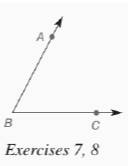Chapter 7.CR, Problem 7CR### Elementary Geometry for College St...

6th Edition
Daniel C. Alexander + 1 other
ISBN: 9781285195698

#### Solutions

Chapter
Section### Elementary Geometry for College St...

6th Edition
Daniel C. Alexander + 1 other
ISBN: 9781285195698
Textbook Problem
1 views

# In Review Exercises 7 to 13, sketch and describe the locus in a plane.Find the locus of points equidistant from the sides of ∠ A B C .To determine

To find: The locus of points equidistant from the sides of ABC.

Explanation

Given:

The ABC is shown below

Theorem used:

The locus of points in a plane and equidistant from the sides of an angle is the angle bisector.

Calculation:

Consider the given figure

By the theorem, we get the locus as the angle bisector as shown below

From, the figure we see that BD bisects ABC

### Still sussing out bartleby?

Check out a sample textbook solution.

See a sample solution

#### The Solution to Your Study Problems

Bartleby provides explanations to thousands of textbook problems written by our experts, many with advanced degrees!

Get Started

#### Evaluate the integrals in Problems 5-28. Check your answers by differentiating. 16.

Mathematical Applications for the Management, Life, and Social Sciences

#### Calculate y'. 24. y=1/x+x3

Single Variable Calculus: Early Transcendentals, Volume I

#### f(x) = x3 2x2 + x 1 has a local maximum at: a) 0 b) 1 c) 13 d) f has no local maxima

Study Guide for Stewart's Single Variable Calculus: Early Transcendentals, 8th

#### Graph: f(x)=x29x21

College Algebra (MindTap Course List)